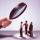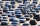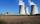Employees

There are 1116 people working in three factory halls. In the first one, there are 18% more than the third, and 60 persons more than the second. How many employees work in individual halls?

Result

a =  413
b =  353
c =  350

Solution:

a+b+c=1116
a = 1.18 * c
a = 60+b

a+b+c = 1116
a-1.18c = 0
a-b = 60

a = 413
b = 353
c = 350

Calculated by our linear equations calculator.

Leave us a comment of this math problem and its solution (i.e. if it is still somewhat unclear...):Be the first to comment!To solve this verbal math problem are needed these knowledge from mathematics:

Our percentage calculator will help you quickly calculate various typical tasks with percentages. Do you have a linear equation or system of equations and looking for its solution? Or do you have quadratic equation?

Next similar examples:

1. Company and employeesThere are 370 employees in the company - women are 15% less than men. How many men work in the company?
2. Enterprise company30% of all employees in the enterprise are women. Men are 360 more. How many employees are in the company?
3. Three workshopsThere are 2743 people working in three workshops. In the second workshop works 140 people more than in the first and in third works 4.2 times more than the second one. How many people work in each workshop?
4. ChildrenThe group has 42 children. There are 4 more boys than girls. How many boys and girls are in the group?
5. RewardThree workers have shared a common reward 13110 CZK follows: first worker got 35% less than the second and third worker got 20% more than the second worker. How much got each worker?
6. Theorem proveWe want to prove the sentence: If the natural number n is divisible by six, then n is divisible by three. From what assumption we started?
7. Three unknownsSolve the system of linear equations with three unknowns: A + B + C = 14 B - A - C = 4 2A - B + C = 0
8. 3 daysWorker checked 2,950 products in 3 days. Second day checked 25% more than the first day. The third day 15% more products than the second day. How many products he checked in each day.
9. SummerjobThe temporary workers planted new trees. Of the total number of 500 seedlings, they managed to plant 426. How many percents did they meet the daily planting limit?
10. Elimination methodSolve system of linear equations by elimination method: 5/2x + 3/5y= 4/15 1/2x + 2/5y= 2/15
11. Profit gainIf 5% more is gained by selling an article for Rs. 350 than by selling it for Rs. 340, the cost of the article is:
12. Ball gameRichard, Denis and Denise together scored 932 goals. Denis scored 4 goals over Denise but Denis scored 24 goals less than Richard. Determine the number of goals for each player.
13. LegsCancer has 5 pairs of legs. The insect has 6 legs. 60 animals have a total of 500 legs. How much more are cancers than insects?55%+36%+88%+71%+100=63% what is whole (X)? Percents can be added directly together if they are taken from the same whole, which means they have the same base amount. .. . You would add the two percentages to find the total amount.Solve two equations with two unknowns: 400x+120y=147.2 350x+200y=144John bought a car for a certain sum of money. He spent 10% of the cost to repairs and sold the car for a profit of Rs. 11000. How much did he spend on repairs if he made a profit of 20%?Last year Karol family reduced its electricity consumption by 31% compared to the previous year and paid CZK 2883 less. How many CZK is electricity last year and how many two year ago?